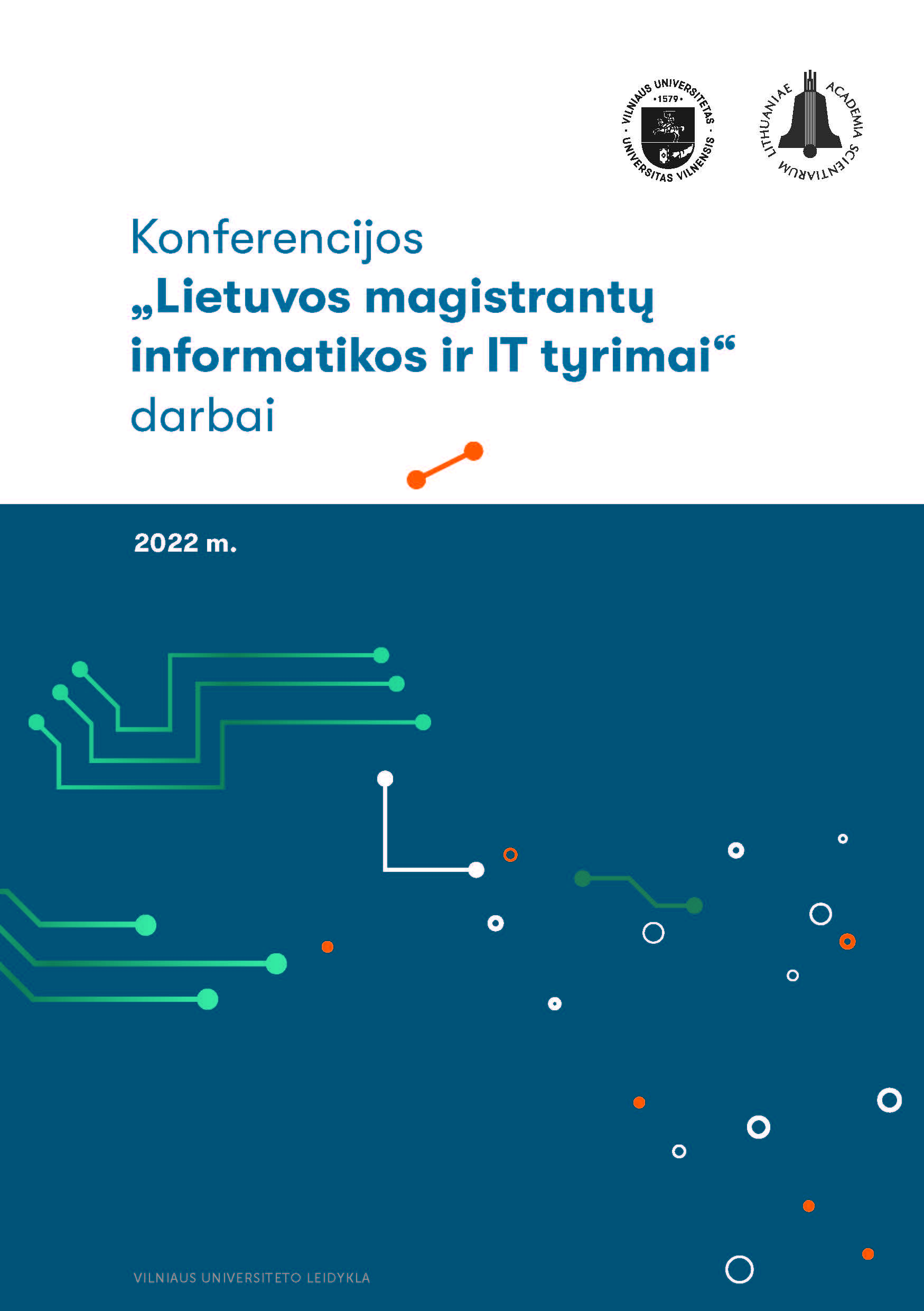Precalculated arrays-based algorithms for the calculation of the Riemann zeta-functionArticles
Lukas Kuzma
Vilnius University, Lithuania
Igoris Belovas
Vilnius University, Lithuania
Martynas Sabaliauskas
Vilnius University, Lithuania
Published 2022-05-13

## Keywords

Riemann zeta function
numerical algorithms
fractal structures

## How to Cite

Kuzma, L., Belovas, I. and Sabaliauskas, M. (trans.) (2022) “Precalculated arrays-based algorithms for the calculation of the Riemann zeta-function”, Vilnius University Open Series, pp. 44–53. doi:10.15388/LMITT.2022.5.

## Abstract

In this paper, we continue the study of efficient algorithms for the computation of the Riemann zeta function on the complex plane. We introduce two precalculated arrays-based modifications of MB-method. We perform numerical experiments with these algorithms using Zetafast as a benchmark and apply the algorithms for the visualizations of fractal structures associated with the Riemann zeta function.